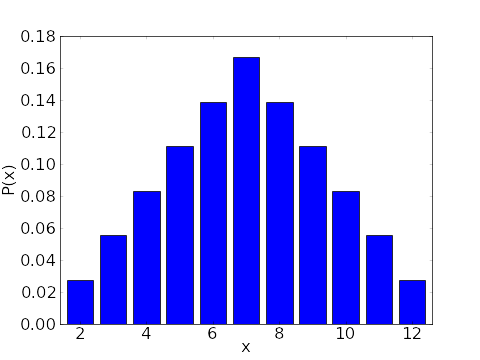# Math Insight

### Image: Probability distribution for the sum of two six-sided diceA bar chart illustrating the probability distribution for a random variable $X$ that is given by the sum of the result of rolling two six-sided dice.

The probability distribution is \begin{gather*} P(x) = \begin{cases} \frac{1}{36} & \text{if $x \in \{2,12\}$}\\ \frac{2}{36}=\frac{1}{18} & \text{if $x \in \{3,11\}$}\\ \frac{3}{36}=\frac{1}{12} & \text{if $x \in \{4,10\}$}\\ \frac{4}{36}=\frac{1}{9} & \text{if $x \in \{5,9\}$}\\ \frac{5}{36} & \text{if $x \in \{6,8\}$}\\ \frac{6}{36} =\frac{1}{6} & \text{if $x = 7$}\\ 0 & \text{otherwise.} \end{cases} \end{gather*}

Image file: two_dice_distribution.png

Source image file: two_dice_distribution.py
Source image type: Python